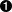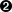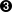Vous devez avoir un compte Developpez.com et être connecté pour pouvoir participer aux discussions.

##### Identifiez-vous
 Identifiant Mot de passe Mot de passe oublié ?
##### Créer un compte

Vous n'avez pas encore de compte Developpez.com ? L'inscription est gratuite et ne vous prendra que quelques instants !Developpez.com

Python

Choisissez la catégorie, puis la rubrique :

17.5. plural.py, stage 4

## 17.5. plural.py, stage 4

Let's factor out the duplication in the code so that defining new rules can be easier.

### Example 17.9. plural4.py

```
import re

def buildMatchAndApplyFunctions((pattern, search, replace)):
matchFunction = lambda word: re.search(pattern, word)applyFunction = lambda word: re.sub(search, replace, word)return (matchFunction, applyFunction)```buildMatchAndApplyFunctions is a function that builds other functions dynamically. It takes pattern, search and replace (actually it takes a tuple, but more on that in a minute), and you can build the match function using the lambda syntax to be a function that takes one parameter (word) and calls re.search with the pattern that was passed to the buildMatchAndApplyFunctions function, and the word that was passed to the match function you're building. Whoa.Building the apply function works the same way. The apply function is a function that takes one parameter, and calls re.sub with the search and replace parameters that were passed to the buildMatchAndApplyFunctions function, and the word that was passed to the apply function you're building. This technique of using the values of outside parameters within a dynamic function is called closures. You're essentially defining constants within the apply function you're building: it takes one parameter (word), but it then acts on that plus two other values (search and replace) which were set when you defined the apply function.Finally, the buildMatchAndApplyFunctions function returns a tuple of two values: the two functions you just created. The constants you defined within those functions (pattern within matchFunction, and search and replace within applyFunction) stay with those functions, even after you return from buildMatchAndApplyFunctions. That's insanely cool.

If this is incredibly confusing (and it should be, this is weird stuff), it may become clearer when you see how to use it.

### Example 17.10. plural4.py continued

```patterns = \
(
('[sxz]\$', '\$', 'es'),
('[^aeioudgkprt]h\$', '\$', 'es'),
('(qu|[^aeiou])y\$', 'y\$', 'ies'),
('\$', '\$', 's')
)rules = map(buildMatchAndApplyFunctions, patterns)```Our pluralization rules are now defined as a series of strings (not functions). The first string is the regular expression that you would use in re.search to see if this rule matches; the second and third are the search and replace expressions you would use in re.sub to actually apply the rule to turn a noun into its plural.This line is magic. It takes the list of strings in patterns and turns them into a list of functions. How? By mapping the strings to the buildMatchAndApplyFunctions function, which just happens to take three strings as parameters and return a tuple of two functions. This means that rules ends up being exactly the same as the previous example: a list of tuples, where each tuple is a pair of functions, where the first function is the match function that calls re.search, and the second function is the apply function that calls re.sub.

I swear I am not making this up: rules ends up with exactly the same list of functions as the previous example. Unroll the rules definition, and you'll get this:

### Example 17.11. Unrolling the rules definition

```rules = \
(
(
lambda word: re.search('[sxz]\$', word),
lambda word: re.sub('\$', 'es', word)
),
(
lambda word: re.search('[^aeioudgkprt]h\$', word),
lambda word: re.sub('\$', 'es', word)
),
(
lambda word: re.search('[^aeiou]y\$', word),
lambda word: re.sub('y\$', 'ies', word)
),
(
lambda word: re.search('\$', word),
lambda word: re.sub('\$', 's', word)
)
)
```

### Example 17.12. plural4.py, finishing up

```
def plural(noun):
for matchesRule, applyRule in rules:if matchesRule(noun):
return applyRule(noun)
```Since the rules list is the same as the previous example, it should come as no surprise that the plural function hasn't changed. Remember, it's completely generic; it takes a list of rule functions and calls them in order. It doesn't care how the rules are defined. In stage 2, they were defined as seperate named functions. In stage 3, they were defined as anonymous lambda functions. Now in stage 4, they are built dynamically by mapping the buildMatchAndApplyFunctions function onto a list of raw strings. Doesn't matter; the plural function still works the same way.

Just in case that wasn't mind-blowing enough, I must confess that there was a subtlety in the definition of buildMatchAndApplyFunctions that I skipped over. Let's go back and take another look.

### Example 17.13. Another look at buildMatchAndApplyFunctions

```
def buildMatchAndApplyFunctions((pattern, search, replace)):```Notice the double parentheses? This function doesn't actually take three parameters; it actually takes one parameter, a tuple of three elements. But the tuple is expanded when the function is called, and the three elements of the tuple are each assigned to different variables: pattern, search, and replace. Confused yet? Let's see it in action.

### Example 17.14. Expanding tuples when calling functions

```>>> def foo((a, b, c)):
...     print c
...     print b
...     print a
>>> parameters = ('apple', 'bear', 'catnap')
>>> foo(parameters)catnap
bear
apple
```The proper way to call the function foo is with a tuple of three elements. When the function is called, the elements are assigned to different local variables within foo.

Now let's go back and see why this auto-tuple-expansion trick was necessary. patterns was a list of tuples, and each tuple had three elements. When you called map(buildMatchAndApplyFunctions, patterns), that means that buildMatchAndApplyFunctions is not getting called with three parameters. Using map to map a single list onto a function always calls the function with a single parameter: each element of the list. In the case of patterns, each element of the list is a tuple, so buildMatchAndApplyFunctions always gets called with the tuple, and you use the auto-tuple-expansion trick in the definition of buildMatchAndApplyFunctions to assign the elements of that tuple to named variables that you can work with.Contacter le responsable de la rubrique Python

Partenaire : Hébergement Web